### LINCON Statement

LINCON l_con [ , l_con …] ;

where l_con is given in one of the following formats:

• linear_term operator number

• number operator linear_term

and linear_term is of the following form: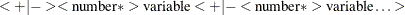The value of operator can be one of the following: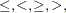or.

The LINCON statement specifies equality or inequality constraints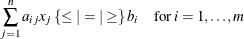separated by commas. For example, the constraint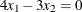is expressed as

   decvar x1 x2;
lincon 4 * x1 - 3 * x2 = 0;


and the constraints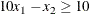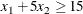are expressed as

   decvar x1 x2;
lincon 10 <= 10 * x1 - x2,
x1 + 5 * x2 >= 15;